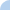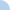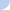What is a solution?

You may take this quiz only two times, so work carefully! This is worth 7 points. Remember that a solution is a number or numbers that make a statement (equation or inequality) TRUE.

 This quiz is timed. The total time allowed for this quiz is 1 hour.Quiz Log In